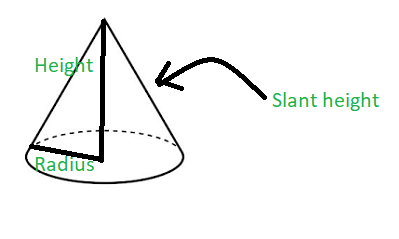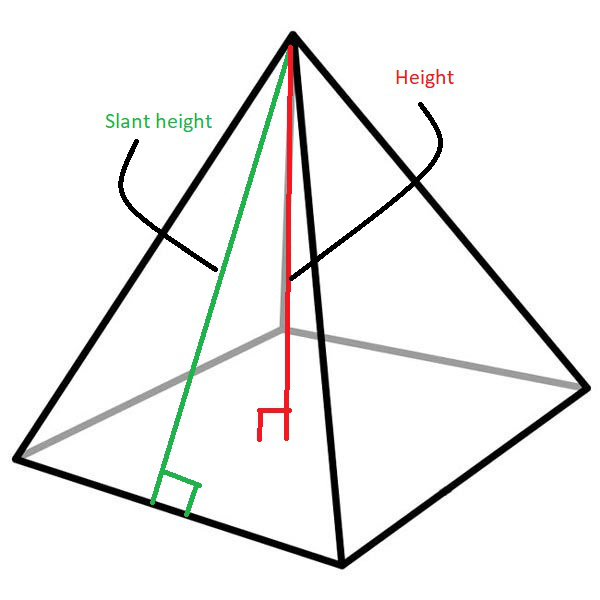# Program to find slant height of cone and pyramid

• Difficulty Level : Easy
• Last Updated : 07 Aug, 2022

Given two integers H1 and R representing the height and radius of a cone and two integers H2 and S representing the height and length of base of a pyramid, the task is to find the slant height of the cone and the pyramid.

Examples:

Input: H1 = 4.5, R = 6, H2 = 4, S = 4.8
Output:
Slant height of cone is: 7.5
Slant height of pyramid is: 4.66476

Input: H1 = 2, R = 4, H2 = 4, S = 8
Output:
Slant height of cone is: 4.47214
Slant height of pyramid is: 5.65685

Approach: The slant height of an object such as a cone or a pyramid is the distance measured from any vertex along a lateral face to the base (along the center of the face). The slant height of a right circular cone is uniform throughout the surface and is given by the formula:where,
L is the slant height of the right circular cone
R is the radius of the right circular cone and
H is the height of the right circular cone

The slant height of a pyramid is given by the formula:where,
L is the slant height of the pyramid
S is the side of the base of the pyramid
H is the height of the pyramid

Below is the implementation of the above approach:

## C++

 // C++ program for the above approach#include using namespace std; // Function to calculate slant// height of a conevoid coneSlantHeight(double cone_h,                     double cone_r){    // Store the slant height of cone    double slant_height_cone        = sqrt(pow(cone_h, 2)               + pow(cone_r, 2));     // Print the result    cout << "Slant height of cone is: "         << slant_height_cone << '\n';} // Function to find the slant// height of a pyramidvoid pyramidSlantHeight(double pyramid_h,                        double pyramid_s){     // Store the slant height of pyramid    double slant_height_pyramid        = sqrt(pow(pyramid_s / 2, 2)               + pow(pyramid_h, 2));     // Print the result    cout << "Slant height of pyramid is: "         << slant_height_pyramid << '\n';} // Driver Codeint main(){    // Dimensions of Cone    double H1 = 4.5, R = 6;     // Function Call for slant height    // of Cone    coneSlantHeight(H1, R);     // Dimensions of Pyramid    double H2 = 4, S = 4.8;     // Function to calculate    // slant height of a pyramid    pyramidSlantHeight(H2, S);     return 0;}

## Java

 // Java program for the above approachimport java.io.*;class GFG{         // Function to calculate slant    // height of a cone    static void coneSlantHeight(double cone_h,                         double cone_r)    {               // Store the slant height of cone        double slant_height_cone            = Math.sqrt(Math.pow(cone_h, 2)                   + Math.pow(cone_r, 2));             // Print the result        System.out.println("Slant height of cone is: " +        slant_height_cone);    }         // Function to find the slant    // height of a pyramid    static void pyramidSlantHeight(double pyramid_h,                            double pyramid_s)    {             // Store the slant height of pyramid        double slant_height_pyramid            = Math.sqrt(Math.pow(pyramid_s / 2, 2)                   + Math.pow(pyramid_h, 2));             // Print the result        System.out.println("Slant height of pyramid is: " +        slant_height_pyramid);    }         // Driver Code    public static void main (String[] args)    {               // Dimensions of Cone        double H1 = 4.5, R = 6;             // Function Call for slant height        // of Cone        coneSlantHeight(H1, R);             // Dimensions of Pyramid        double H2 = 4, S = 4.8;             // Function to calculate        // slant height of a pyramid        pyramidSlantHeight(H2, S);         }} // This code is contributed by AnkThon

## Python3

 # Python 3 program for the above approachfrom math import sqrt,pow# Function to calculate slant# height of a conedef coneSlantHeight(cone_h, cone_r):  # Store the slant height of cone  slant_height_cone = sqrt(pow(cone_h, 2) + pow(cone_r, 2))   # Print the result  print("Slant height of cone is:",slant_height_cone) # Function to find the slant# height of a pyramiddef pyramidSlantHeight(pyramid_h, pyramid_s):  # Store the slant height of pyramid  slant_height_pyramid = sqrt(pow(pyramid_s/2, 2) + pow(pyramid_h, 2))    # Print the result  print("Slant height of pyramid is:","{:.5f}".format(slant_height_pyramid)) # Driver Codeif __name__ == '__main__':  # Dimensions of Cone  H1 = 4.5  R = 6   # Function Call for slant height  # of Cone  coneSlantHeight(H1, R);   # Dimensions of Pyramid  H2 = 4  S = 4.8   # Function to calculate  # slant height of a pyramid  pyramidSlantHeight(H2, S)

## C#

 // C# program for the above approachusing System;public class GFG{   // Function to calculate slant  // height of a cone  static void coneSlantHeight(double cone_h,                              double cone_r)  {     // Store the slant height of cone    double slant_height_cone      = Math.Sqrt(Math.Pow(cone_h, 2)                  + Math.Pow(cone_r, 2));     // Print the result    Console.WriteLine("Slant height of cone is: " +                      slant_height_cone);  }   // Function to find the slant  // height of a pyramid  static void pyramidSlantHeight(double pyramid_h,                                 double pyramid_s)  {     // Store the slant height of pyramid    double slant_height_pyramid      = Math.Sqrt(Math.Pow(pyramid_s / 2, 2)                  + Math.Pow(pyramid_h, 2));     // Print the result    Console.WriteLine("Slant height of pyramid is: " +                      slant_height_pyramid);  }   // Driver Code  public static void Main (string[] args)  {     // Dimensions of Cone    double H1 = 4.5, R = 6;     // Function Call for slant height    // of Cone    coneSlantHeight(H1, R);     // Dimensions of Pyramid    double H2 = 4, S = 4.8;     // Function to calculate    // slant height of a pyramid    pyramidSlantHeight(H2, S);   }} // This code is contributed by AnkThon

## Javascript

 

Output:

Slant height of cone is: 7.5
Slant height of pyramid is: 4.66476

Time Complexity: O(sqrt(logH1+logR) + sqrt(logH2+logS))
Auxiliary Space: O(1)

My Personal Notes arrow_drop_up